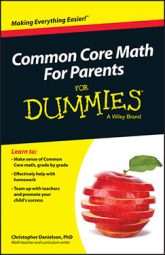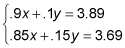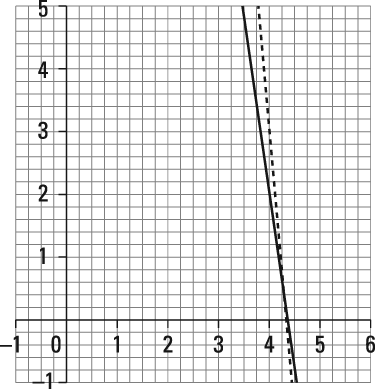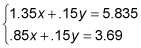##### Common Core Math For Parents For Dummies with Videos OnlineIn Common Core math, eighth graders write and solve systems of linear equations. They use symbolic techniques and read approximate solutions from graphs. A system of equations in eighth grade is a set of two equations, each using the same two variables, and the constraint that the same values for these variables must solve both equations.

For example, say your local butcher shop sells 90 percent lean ground beef for \$3.89 per pound and 85 percent lean ground beef for \$3.79 per pound. If you let x be the price per pound of purely lean beef and y be the price per pound of pure beef fat, then you can write the following system of linear equations (the curly bracket on the left indicates that these two equations go together and that you're interested in any x and y values that make both equations true at the same time):Writing a system of equations such as this one requires making a bunch of assumptions about the real world. For example, you have to assume that there is such a thing as purely lean beef and that the butcher store would sell it to you. You also have to assume that ground beef is made by mixing purely lean beef together with pure beef fat and so on. Lots of assumptions — but even if these assumptions aren't all valid, the mathematical solution to the system still gives a starting point for talking about how these pricing decisions get made.A graphical solution to a system of equations.

In this case, you can read an approximate solution off the graph in the figure by noticing where the two lines cross each other. Purely lean beef should be priced at \$4.29 per pound and pure beef fat at \$0.29 per pound.

Here is a brief description of a symbolic technique for solving this system of equations. Imagine you bought 1.5 pounds of 90 percent lean ground beef. Then the first equation in the system changes to show that you have 1.35 pounds of purely lean beef, 0.15 pounds of pure beef fat, and that you would pay \$5.835. The new system of equations looks like this:Both of these equations involve 0.15 pounds of pure beef fat, which means that the additional cost of the 90 percent lean ground beef (the 5.835 in the top equation) comes only from the additional purely lean beef. This additional purely lean beef is half a pound (the difference between 0.85 and 1.35 pounds). You can write this new relationship as 0.5x = 2.145 (the additional half pound of purely lean beef costs \$2.145). You can solve for x to get x = 4.29. This matches the value you can see on the graph. This kind of thinking develops into the algebraic technique of elimination. Eighth graders solve systems of linear equations by elimination as well as by working with graphs.